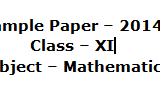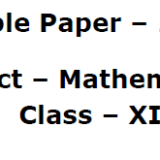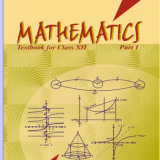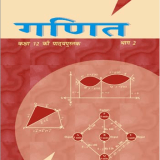Home » Science » Archive by category "Mathematics"

## Sample Paper of Mathematics 2014 for class 11, CBSE. Paper No.2Sample Paper – 2014 Class – XI Subject – Mathematics Maximum Time– 3 Hrs                                                                                 Maximum Marks – 100   General instructions – All questions are compulsory. The question paper consists of 29 questions divided in to three sections A, B and C. Section A comprises of 10 questions of 1 mark each, Section B comprises of 12 questions of 4 marks each and Section C comprises of 7 questions of...

## Sample Paper of Mathematics 2014 for class 11, CBSE. Paper No.1Sample Paper – 2014 Class – XI Subject – Mathematics Time: 3Hrs.                                                                                                                  Max. Marks: 100 General Instructions: (The question paper has two printed pages divided in 29 questions as under) All the questions are compulsory. The question paper has been divided in 3 sections A, B and C. Section A contains 10 questions of 1 mark each. Section B contains 12 questions of 4 marks...

## Sample Paper of Mathematics “( Board Mock Test) – 2010″for class 12, CBSE. Paper No.2, PDFmcm online store boxing day gucci prada outlet uk michael kors uk handbags mcm bags for sale mcm tote bag alviero martini portafogli sac hermes kelly cheap timberland boots cheap mulberry bags Related posts: Sample Paper of Mathematics 2014 for class 12, CBSE. Paper No.1, PDF Sample Paper of Mathematics 2014 for class 11, CBSE. Paper No.1 Sample Paper of Mathematics 2014 for class 11, CBSE. Paper No.2 Mathematics (041) CBSE...

## Sample Paper of Mathematics 2014 for class 12, CBSE. Paper No.1, PDFtoms after christmas sale mcm online store mulberry sale uk michael kors handbags sac kelly hermes toms wedges mcm purse alviero martini portafogli alviero martini shop online cheap pradas Related posts: Sample Paper of Mathematics “( Board Mock Test) – 2010″for class 12, CBSE. Paper No.2, PDF Mathematics (041) CBSE Question Papers 2015-2016, Complete Question Papers of Mathematics (041) CBSE.

## Mathematics  text book “Mathematics part 2” ebook for class 12, CBSE, NCERTChapter1.pdf Chapter2.pdf Chapter3.pdf Chapter4.pdf Chapter5.pdf Chapter6.pdf Prelims.pdf Related posts: Mathematics text book “Exemplar Problem” English medium ebook for class 12, CBSE, NCERT Mathematics  text book “Exemplar Problem” Hindi medium ebook for class 12, CBSE, NCERT Mathematics  text book “Ganit part 2” Hindi medium ebook for class 12, CBSE, NCERT Mathematics  text book “Mathematics part 1” ebook for class 12, CBSE, NCERT   Mathematics text book “Ganit part 1” Hindi medium ebook...

## Mathematics  text book “Mathematics part 1” ebook for class 12, CBSE, NCERTChapter1.pdf Chapter2.pdf Chapter3.pdf Chapter4.pdf Chapter5.pdf Chapter6.pdf prelims.pdf Related posts: Mathematics text book “Exemplar Problem” English medium ebook for class 12, CBSE, NCERT Mathematics  text book “Exemplar Problem” Hindi medium ebook for class 12, CBSE, NCERT Mathematics  text book “Ganit part 2” Hindi medium ebook for class 12, CBSE, NCERT Mathematics  text book “Mathematics part 2” ebook for class 12, CBSE, NCERT   Mathematics text book “Ganit part 1” Hindi medium ebook...